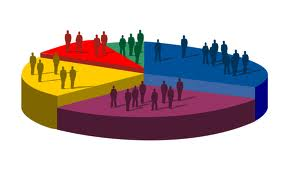# Types of StatisticsStatistical methods can be used to summarize or describe a collection of data; this is called descriptive statistics. This is useful in research, when communicating the results of experiments.

There are two types of statistics

1. Descriptive Statistics
2. Inferential Statistics

1. Descriptive statistics

For many people, statistics means numbers—numerical facts, figures, or information. Reports of industry production, baseball batting averages, government deficits, and so forth, are often called statistics. To be precise, these numbers are descriptive statistics because they are numerical data that describe phenomena. Descriptive statistics are as simple as the number of children in each family along a city block or as complex as the annual report released from the U.S. Treasury Department.

Consider two ways of representing descriptive statistics: numerical and pictorial.

Numerical statistics

Numerical statistics are numbers, but clearly, some numbers are more meaningful than others. For example, if you are offered a purchase price of \$1 for an automobile on the condition that you also buy a second automobile, the price of the second automobile would be a major consideration (its price could be \$1,000,000 or only \$1,000), and thus, the average—or mean—of the two prices would be the important statistic.

Pictorial statistics

Taking numerical data and presenting it in pictures or graphs is what is known as pictorial statistics. Showing data in the form of a graphic can make complex and confusing information appear more simple and straightforward.

2.  Inferential Statistics

Inferential statistics is a decision, estimate, prediction, or generalization about a population, based on sample.

A population is a collection of all possible individual, objects, or measurements of interest.

A sample is a portion, or part, of the population of interest.

With inferential statistics, we are trying to reach conclusions that extend beyond the immediate data alone. For instance, we use inferential statistics to try to infer from the sample data what the population might think. Or, we use inferential statistics to make judgments of the probability that an observed difference between groups is a dependable one or one that might have happened by chance in this study. Thus, we use inferential statistics to make inferences from our data to more general conditions; we use descriptive statistics simply to describe what’s going on in our data.